# High School Physics : Understanding Doppler

## Example Questions

### Example Question #1 : Understanding Doppler

An ambulance turns on its siren as it is driving away from you. What happens to the sound pitch?

There is insufficient information to tell.

The pitch will sound higher.

The pitch will sound lower.

You will be unable to hear the pitch.

The pitch will stay the same.

The pitch will sound lower.

Explanation:

The Doppler effect states that the frequency of sound increases as it approaches you and decreases as it goes away from you.

Since it is going away from you, the pitch of the sound will appear to get lower.

### Example Question #1 : Understanding Doppler

An ambulance turns on its siren as it is driving towards you. What happens to the sound pitch?

The pitch will stay the same.

The pitch will sound higher.

The pitch will sound lower.

You will be unable to hear the pitch.

There is insufficient information to solve.

The pitch will sound higher.

Explanation:

The Doppler effect states that the frequency of sound increases as it approaches you and decreases as it goes away from you.

Since it is coming towards you, the pitch will appear to be higher.

### Example Question #1 : Understanding Doppler

An ambulance drives past a stationary man. Its siren has a frequency of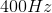. As the ambulance passes the man and drives off, which of the following is a possible frequency that the man would hear?

We would need to know the velocity of the ambulance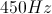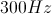We would need to know how far the man is from the ambulance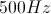Explanation:

When the ambulance passes the man, there is blue shift according to the Doppler effect. That means that the perceived frequency will be lower than the given one. Only one answer choice has a lower observed frequency than the actual frequency: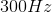.

### Example Question #651 : High School Physics

An ambulance drives past a stationary man. Its siren has a frequency of. As the ambulance approaches the man, which of the following is a possible frequency that the man would hear?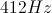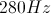We would need to know the distance the man is from the ambulanceWe would need to know the speed of the ambulanceExplanation:

When the ambulance approaches the man, there is red shift according to the Doppler effect. That means that as it approaches, the observed frequency increases. There is only one answer choice with a higher observed frequency than the actual frequency: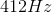.

### Example Question #1 : Sound

You are standing on a street corner when an ambulance rushes past you, blaring its siren. At what point do you actually hear the frequency that the ambulance siren is emitting?

You can never hear the actual frequency, since the ambulance is moving

When it is right in front of you

When it is moving away from you

When it is moving towards you

Throughout the entire motion of the ambulance

When it is right in front of you

Explanation:

The Doppler effect states that the frequency of sound shifts proportionally to its velocity, either moving towards you or away from you. As it moves towards you, the perceived frequency increases. As it moves away, the perceived frequency decreases. The only time you will hear the correct frequency is when the observer and sound source are directly aligned, so that the frequency is neither increased nor decreased.

### Example Question #3 : Understanding Doppler

You and a friend are running down the street at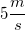. Your friend yells something. What will happen to the frequency of the yell that you perceive, compared to the sound that your friend actually made?

The frequency of the sound pulses between higher and lower levels

We must know the distance between the source and the observer to solve

There is no change in the frequency

The frequency of the sound goes up

The frequency of the sound goes down

There is no change in the frequency

Explanation:

Change in frequency due to relative motion is described by the Doppler effect, given by the equation: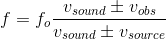The numerator terms are summed when the observer is moving toward the source. The denominator terms are summed if the source is moving away from the observer. You and your friend are both moving with the same velocity in the same direction, meaning that the source (friend) is moving toward the observer (you) AND the observer (you) is moving away from the source (friend). The result is that both the numerator and denominator terms will be subtracted.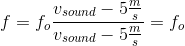The fraction simplified to one. The perceived frequency is equal to the transmitted frequency.

### All High School Physics Resources